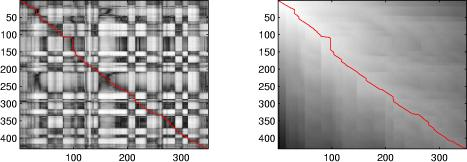Dan Ellis : Resources : Matlab :

# Dynamic Time Warp (DTW) in Matlab

#### Introduction

One of the difficulties in speech recognition is that although different recordings of the same words may include more or less the same sounds in the same order, the precise timing - the durations of each subword within the word - will not match. As a result, efforts to recognize words by matching them to templates will give inaccurate results if there is no temporal alignment.

Although it has been largely superseded by hidden Markov models, early speech recognizers used a dynamic-programming technique called Dynamic Time Warping (DTW) to accommodate differences in timing between sample words and templates. The basic principle is to allow a range of 'steps' in the space of (time frames in sample, time frames in template) and to find the path through that space that maximizes the local match between the aligned time frames, subject to the constraints implicit in the allowable steps. The total `similarity cost' found by this algorithm is a good indication of how well the sample and template match, which can be used to choose the best-matching template.

The code and example on this page show a simple implementation of dynamic time warp alignment between soundfiles. In addition to using this for scoring the similarity between sounds, we can also use it to `warp' a soundfile to match the timing of a reference, for instance to synchronize two utterances of the same words.

#### Code

The routines provided here are:

• simmx.m - a utility to calculate the full local-match matrix i.e. calculating the distance between every pair of frames from the sample and template signals.
• dp.m - implementation of the simple dynamic programming algorithm that allows three steps - (1,1), (0,1) and (1,0) - with equal weights.
• dp2.m - experimental alternative version that allows 5 steps - (1,1), (0,1), (1,0), (1,2), and (2,1) - with different weights to prefer sloping paths but without a hard limit on regions in which matches are found. Seems to work better, but not much tested. Syntax etc. the same as dp.m.
• dpfast.m - fast version that uses a MEX routine (dpcore) to execute the non-vectorizable inner loop - as much as 200 times faster! Also allows user-specified step/cost matrix.
• dpcore.c - C source for the MEX routine that speeds up dpfast.m. Also available as precompiled MEX extensions for Mac OS X: dpcore.mexmac, dpcore.mexmaci, dpcore.mexmaci64, and Linux: dpcore.mexglx, dpcore.mexa64.

#### Example

An example use is shown below:

```% Load two speech waveforms of the same utterance (from TIMIT)[d1,sr] = wavread('sm1_cln.wav');[d2,sr] = wavread('sm2_cln.wav');% Listen to them together:ml = min(length(d1),length(d2));soundsc(d1(1:ml)+d2(1:ml),sr)% or, in stereosoundsc([d1(1:ml),d2(1:ml)],sr)% Calculate STFT features for both sounds (25% window overlap)D1 = specgram(d1,512,sr,512,384);D2 = specgram(d2,512,sr,512,384);% Construct the 'local match' scores matrix as the cosine distance% between the STFT magnitudesSM = simmx(abs(D1),abs(D2));% Look at it:subplot(121)imagesc(SM)colormap(1-gray)% You can see a dark stripe (high similarity values) approximately% down the leading diagonal.% Use dynamic programming to find the lowest-cost path between the% opposite corners of the cost matrix% Note that we use 1-SM because dp will find the *lowest* total cost[p,q,C] = dp(1-SM);% Overlay the path on the local similarity matrixhold on; plot(q,p,'r'); hold off% Path visibly follows the dark stripe% Plot the minimum-cost-to-this point matrix toosubplot(122)imagesc(C)hold on; plot(q,p,'r'); hold off% Bottom right corner of C gives cost of minimum-cost alignment of the twoC(size(C,1),size(C,2))
ans =
128.2873% This is the value we would compare between different% templates if we were doing classification.% Calculate the frames in D2 that are indicated to match each frame% in D1, so we can resynthesize a warped, aligned versionD2i1 = zeros(1, size(D1,2));for i = 1:length(D2i1); D2i1(i) = q(min(find(p >= i))); end% Phase-vocoder interpolate D2's STFT under the time warpD2x = pvsample(D2, D2i1-1, 128);% Invert it back to time domaind2x = istft(D2x, 512, 512, 128);% Listen to the results% Warped version alonesoundsc(d2x,sr)% Warped version added to original target (have to fine-tune length)d2x = resize(d2x', length(d1),1);soundsc(d1+d2x,sr)% .. and in stereosoundsc([d1,d2x],sr)% Compare to unwarped pair:soundsc([d1(1:ml),d2(1:ml)],sr)
```

#### Caveat - negative costs

The figure below shows two different DP paths through the underlying cost matrix. In the green trace, we see horizontal and vertical steps being taken in preference to the diagonal steps in the red trace. This is a symptom of having negative cost values - e.g. using [p,q] = dpfast(-M) instead of [p,q] = dpfast(1-M).

DP finds the path that minimizes the total cost. With positive costs, that means finding both the low-cost cells, and minimizing the total number of steps.

With negative costs, the path still seeks out the lowest (in this case most negative) cost cells. But it also rewards *longer* paths, since accumulating a greater number of negative costs gives a lower total cost. Since [across, down] is two steps compared to a single diagonal step, negative costs will lead to this kind of stairstep path and few diagonal steps. But diagonal steps (and a cost matrix that is never less than zero) is most likely what you want.#### Referencing

If you find this code useful and wish to reference it in your publications, you can make a reference directly to this web page, e.g. something like:

D. Ellis (2003). Dynamic Time Warp (DTW) in Matlab
Web resource, available: http://www.ee.columbia.edu/~dpwe/resources/matlab/dtw/.

The code originated with our project on aligning MIDI descriptions to music audio recordings,

R. Turetsky and D. Ellis (2003)
Ground-Truth Transcriptions of Real Music from Force-Aligned MIDI Syntheses
4th International Symposium on Music Information Retrieval ISMIR-03, pp. 135-141, Baltimore, October 2003.